## What are extended chords?

We can consider as extended chords those chords that have tension notes (or notes of available tension).

## What are tension notes?

Tension notes are the other notes that form a chord, in addition to the so-called chord notes (or chord tones). Remember that chord notes are those that form the triad or tetrad of the chord.

Let’s take the Cmaj7 chord as an example. It is formed by the notes C, E, G, B, which correspond to degrees 1, 3, 5 and 7. This is the tetrad of this chord, that is, the notes C, E, G, B are the so-called “chord notes” of Cmaj7.

If we added any notes to that chord, for example, the ninth, the chord would be: Cmaj7(9). In this case, the ninth would be called a “tension note“, this terminology is widely used in musical theory literature.

All notes other than the 1st, 3rd, 5th and 7th degrees will be called tension notes. Notice then that there are only 3 possible degrees of tension (the fourth, the sixth and the ninth). Note: the ninth is equivalent to the second degree.

So far, we’ve only used the tetrad to form a tonality (we talked about Cmaj7, Dm7, etc.). Then, to conclude this subject, it is time to analyze the remaining notes (4th, 6th and 9th). These notes, when added, end up making the extended chords.

Our study will show which of these notes can be used for each chord within the major key. That is, for a song that is in C major, for example, can I play the Dm6 chord? What about the FM7(9) chord? These questions will all be answered.

This will help you when composing or reharmonizing songs, as you will know which tensions can be used in each chord and which tensions should be avoided (avoid notes).

## What are avoid notes?

Avoided notes are those you should avoid playing. The reasons for avoiding a note are as follows:

We will explain in detail what each one is.

Let’s use the C major key as an example, remembering that these concepts apply to all other notes.

The chords of the C major key are: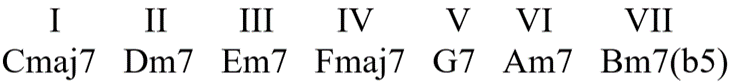When we talk about the notes to be avoided, remember that we are talking about notes that belong to the major scale of C, after all the key is C major.

This is important to highlight because, for example, the scale of the Fmaj7 chord (in this key of C) is F Lydian, not F major. So, for this chord, we will be using the Lydian scale. So don’t be alarmed when you come across an augmented fourth, for example, analyzing whether or not it should be avoided in this case.

We are analyzing only the notes of the C major scale, and these notes, when the chord is not C, receive a different reference from the point of view of the degrees; so you will see diminished fourth, augmented fourth, etc. Think of the musical modes. The major scale shape will be used only for the Cmaj7; the rest of the chords will have their scales according to the respective mode. I suggest that you have the scales of the modes at your side to facilitate your study on this topic.

## The rule to discover avoid notes:

We already know that each note of the major scale has a function. These functions are, respectively: I degree (tonic), IV degree (subdominant), VII degree (leading tone).

Therefore, in the case of C major, we will have the notes: “C” representing the tonic function, “F” representing the subdominant function and “B” representing the dominant function.

To create our rule, we will use the sequence tonic – subdominant – dominant – tonic … repeating the cycle always in that order.

The rule will be as follows: for each chord of the major key, we will avoid playing the note that represents the next function. That is, when the chord has the tonic function, we will avoid playing the note that represents the subdominant function. When the chord has the subdominant function, we will avoid playing the note that represents the dominant function. And so on.

With that rule in mind, we can begin to discover the available tensions for each one of the chords.

### Tensions for the Cmaj7 chord:

We will start by analyzing the first chord (Cmaj7). Observe the C major scale below and see the possible extensions (fourth, sixth and ninth):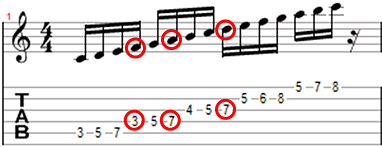The notes are, respectively, F, A and D. Let’s see how the Cmaj7 chord looks with each of these tensions:

With fourth: Cmaj7(4)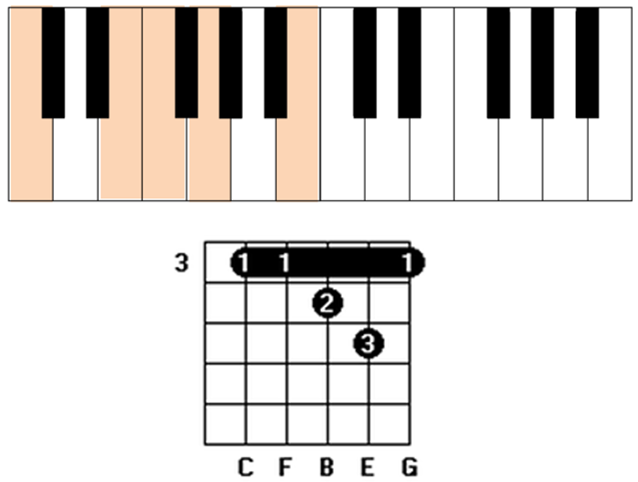With sixth: Cmaj7(13)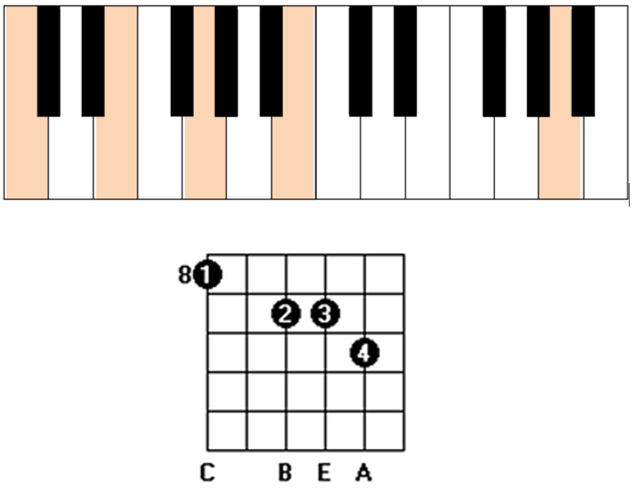With ninth: Cmaj7(9)The question remains: can we use all these tensions within the C major key? Answer: all tensions can be used, except the fourth degree. That is, we cannot play C4 or Cmaj7(4). Reason: in this context, the C chord has a tonic function, and the F note represents the subdominant function, and we should avoid playing the note that represents the next function.

In practice, when placing this note, we end up decharacterizing the tonic function of the C chord, because the F note played with the B note (in the Cmaj7(4) chord) forms a tritone, and we already know that the sound of the tritone is “tense”.

In addition, it is worth noting that the F note is a semitone away from the E note, which is a Cmaj7 chord note (the third). What’s wrong with that?

Well, if we put the F note with the C chord, forming a C4, we will be simultaneously playing two notes that are separated by a semitone (E and F), and the third is the note that defines whether a chord is major or minor. That sounds very unpleasant. Take your instrument and sound two notes that are separated by a semitone simultaneously. Notice how bad it sounds? This is explained by the fact that it is a chromatic approach. You will learn, in the study of “SubV7“, that this approach serves to prepare the way to where we want to arrive.

For example, let’s say that a bass player is playing the G note, within the key of C, because the chord of the moment is G, and the next chord in the song is A minor.

Before playing the A note, the bassist could play G sharp and then play A. This chromatic approach effect sounds very good, as it seems that we are going up a ladder (G, G#, A), where the next step is already indicated (when we touch G sharp, we immediately expect the next note to be A).

For this reason, playing G sharp along with A (both at the same time) produces confusion. The impression it gives is that we are in conflict, as the two notes are very close and should be played in sequence, not at the same time.

The confusion arises from the doubt of our brain: “Do you want to rest in A flat or in A?”, After all, the chromatic sequence could be Ab – A or A – Ab. In the first case, Ab would be a passing note to rest in A (ascending cadence), and in the second case, A would be a passing note to rest in Ab (descending cadence).

But it is important to note that when the notes are in different octaves, this effect is minimized, for example: C11.

Perhaps you are thinking: “But then I will never be able to play a chord with the fourth, after all the fourth is always a semitone away from the third degree (which is a chord note)”.

This reasoning makes sense and is true. But there is a solution: we can take the third degree out of the chord! So there would be no such conflict. Since, in this case, there would be no third degree, the chord is suspended.

Moral of the story: chords with 4th are usually suspended. That’s why you’ll see Asus4, etc. Chords with fourth will have the “sus” indicating that the third degree has been taken way from the chord.

On the other hand, when the fourth is a few octaves away from the major third, this difference in bass and treble helps to minimize the unpleasant effect, mainly because the low notes of the chord are mixed with the low notes and the high notes are mixed with the high notes, causing a separation between a low F and a high E, for example.

However, even if the C chord is suspended in our case (Csus4), despite minimizing the unpleasant effect, it would still decharacterize the tonic function, as it would no longer sound serene and resting, which is expected from a tonic function. Therefore, this note should be considered “avoided” for the first chord of the major key.

## Tensions for the Dm7 chord

Continuing our study of tension notes, we will analyze our next chord of the major key of C: Dm7. Since this chord has a subdominant function, we should avoid the note that characterizes the dominant function (B note). In relation to D, B is the sixth degree, therefore we should avoid playing Dm6. We can see that the Dm6 chord has the same notes as the Bm7(b5) chord, compare them:

• Notes of Dm6 = D, F, A, B
• Notes of Bm7(b5) = B, D, F, A

Therefore, when placing the sixth on the Dm6 chord, it would be sounding as if it were Bm7(b5), which is a dominant function chord (it has a tritone), decharacterizing its function which should be subdominant.

See some Dm7 options with their available tensions below:

With fourth: Dm7(4)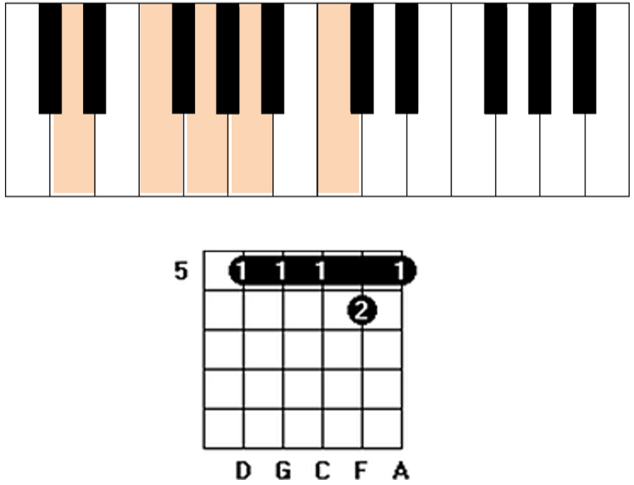With the ninth: Dm7(9)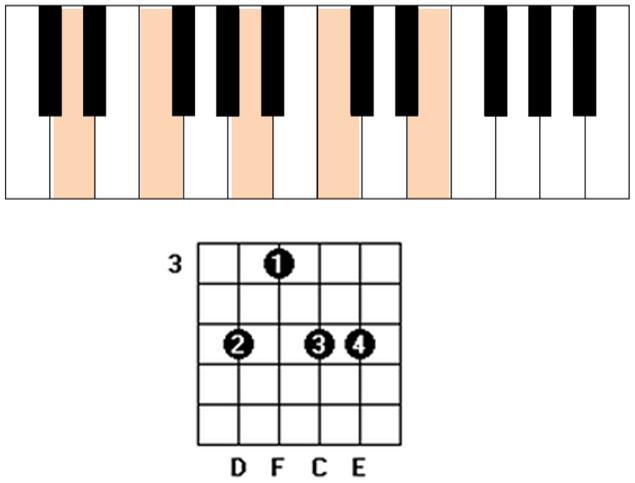## Tensions for the Em7 chord

Blocked content…